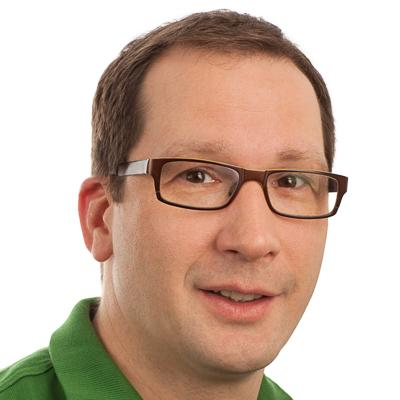# Analysis

The research activities of the analysis group centre around the following areas and the interplay between them:

### Harmonic analysis with applications to PDEs, geometry, and signal analysis

Classical harmonic analysis has its roots in the theory of representations of functions and is considered to be a generalization of Fourier analysis. It includes Calderon-Zygmund theory of singular integrals and Littlewood-Paley theory and also has interesting connections to probability theory. Methods and ideas of harmonic analysis have found numerous applications in the theory of partial differential equations, spectral geometry and signal analysis.

### Several complex variables

Several complex variables deals with the study of complex structures and metric structures on analytic spaces. This area is closely related to convex geometry and algebraic geometry and has also found many applications in symplectic geometry and number theory. In addition, it provides tools such as sheaf theory, Hörmander-Kohn-L2 theory and Kodaira-Spencer deformation theory.

### Complex analysis and operator theory

The interplay between complex analysis and operator theory dates back to a revolutionary paper of Beurling from 1949 and is nurtured by applications in engineering sciences and mathematical physics. This vast area studies spaces of holomorphic functions emerging in various applications and properties of concrete operators and classes of operators acting on functions in such spaces.

### Analytic number theory

Analytic number theory uses methods from analysis to study properties of natural numbers. Of particular interest is the interplay between the additive and multiplicative structure of the integers. A central object of study in this area is the Riemann zeta function which embodies these two structures in a single function. The theory of the Riemann zeta function centres around the famous Riemann hypothesis.

### Operator algebras and noncommutative geometry

Noncommutative geometry extends the natural correspondence between geometric spaces and commutative algebras to the noncommutative case. This allows us to extend the classical tools—such as measure theory, topology, differential calculus and Riemannian geometry—to the noncommutative situation.

## ContactProfessor Franz Luef

## Current projects

Fourier Methods and Multiplicative Analysis

The theory of the Riemann zeta function; multiplicative analysis; Fourier interpolation and quasi-crystals. Funded by FRIPRO. Contact: Kristian Seip

Completed projects